Your data analysis solution

# Association rules for market basket analysis tutorial

2017-10-20

This tutorial will help you set up and interpret association rules learning for market basket analysis in Excel with XLSTAT.

## Association rules mining

In 1994, Rakesh Agrawal and Ramakrishnan Sikrant have proposed an algorithm to identify associations between items in the form of rules. This algorithm is used when the volume of data to be analyzed is important. As the number of items can be several tens of thousands, combinatorics are such that all the rules can not be studied. It is therefore necessary to limit the search for rules to the most important ones. The quality measurements are probabilistic values which limit the combinatorial explosion during the two phases of the algorithm, and allow the sorting of the results.

Definitions:

Items: Depending on the application field, they can be products, objects, patients, events.

Transaction: Identified with a unique identifier, it is a set of items with a minimum of one item. Items can belong to several transactions.

Itemset: A group of items. Itemsets can be found in one or more transactions.

Support: The probability to find item or itemset X in a transaction. Estimated by the number of times an item or itemset is found across all the available transactions. This value lies between 0 and 1.

Rule: A rule defines a relationship between two itemsets X and Y that have no items in common. X->Y means that if we have X in a transaction, then we can have Y in the same transaction.

Support of a rule: The probability to find items or itemsets X and Y in a transaction. Estimated by the number of times both items or itemsets are found across all the available transactions. This value lies between 0 and 1.

Confidence of a rule: The probability to find item or itemset Y in a transaction, knowing item or itemset X is in the transaction. Estimated by the observed corresponding frequency (number of times X and Y are found across all transactions divided by the number of times X is found). This value lies between 0 and 1.

Lift of a rule: The lift of a rule, which is symmetric (Lift(X->Y)=Lift(Y->X)), is the support of the itemset grouping X and Y, divided by the support of X and the support of Y. This value can be any positive real. A lift greater than 1 implies a positive effect of X on Y (or Y on X) and therefore the significance of the rule. A value of 1 means there is no effect and it is as if the items or itemsets are independent. A lift lower than 1, means there is a negative effect of X on Y or reciprocally. As if they were excluding each other.

## Dataset

An Excel sheet with both the data and the results can be downloaded by clicking here. The dataset used in this tutorial is an extract from the dataset that was donated by Tom Brijs (http://fimi.ua.ac.be/data/retail.pdf, T. Brijs, G. Swinnen, K. Vanhoof and G. Wets. The use of association rules for product assortment decisions: a case study. In: Proceedings of the Fifth International Conference on Knowledge Discovery and Data Mining, San Diego (USA), August 15-18, 254-260, 1999). It contains the anonymized retail market basket data from an anonymous Belgian retail store. The original dataset contains 88163 transactions, but we reduced it to the first 65000 transactions so that this tutorial can be used by Excel 2003 users. The association rules tool allows to work as well directly on flat files with gigabites of data, but this example is based on an Excel workbook.

XLSTAT accepts the following layouts for the input data:

• Transactional: Choose this format if your data is in two columns, one indicating the transaction (to be selected in the Transactions field), the other item. Typically with this format, there is a column with the transaction IDs, with for each transaction, as many rows as there are items in the transaction, and a column indicating the items. The transactions can be in the first column and selected in this field.
• List: Choose this format if your data include one line per transaction while columns contain the names of the items corresponding to the transaction. The number of items per transaction may vary from one line to another. The number of columns in the selection corresponds to the maximum number of items per transaction.
• Transactions/Variables: Choose this format if your data correspond to one line per transaction and to one column per variable. This format is such that all transactions have the same number of items, which is the number of variables, and that items from a given variable cannot be present in the same transaction.
• Contingency table: Choose this format if your data include one row per transaction and a column per item, with null values if the item is not present and a number greater than 1 if it is present.

In this tutorial, the data are available in list format, where each row represents one transaction. There are as many columns as items per transaction.

## Setting up the association rules analysis

After opening XLSTAT, select the XLSTAT / Machine Learning / Association rules command, or click on the corresponding button of the "XLSTAT" toolbar.Once you've clicked on the button, the  dialog box will appear. Select the data on the Excel sheet. In the “Items” field, select columns A to BV that contain all the transactions and items (in order to identify the last column containing a transaction, press Ctrl End which moves the cursor to the bottom right end of the dataset). The option Labels included is inactivated because the first row of the selected data does not contain headers.

The minimum support is left to its default value. Rules that have a support lower than this value will not be taken into account.

The minimum confidence is left to its default value. Rules that have a confidence lower than this value will not be taken into account.

The minimum number of antecedents is left to its default value. There is no specific constraint on the number of items contained in the left part (X) of the rule (X->Y).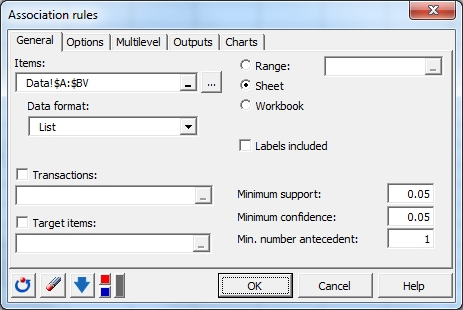In the Options tab, we choose that the rules are sorted based on their confidence.The computations begin once you have clicked the OK button. The computations last for about 35 seconds. A window is displayed so that you can stop the analysis at any time. Then the results are displayed.

## Interpreting the results of an association rules analysis

The first results confirm the number of items in the dataset and the number of transactions. The summary of association rules table displays all the rules that meet the constraints defined in the General tab of the dialog box. We see here that the rule with the highest confidence is the rule that says that if products 41 and 48 are in the basket, then there is 81.6%  chance that there is also product 39. This rule is found in 7.9% of the transactions. The lift is 1.426 which means that having (41 and 48) or respectively (39) increases the chance of having (39) or respectively (41 and 48) by a factor of 1.426.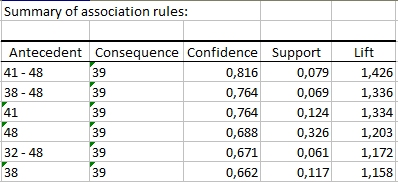The matrix of influence is another way to show the confidences of having the items in columns knowing that the items in rows are present.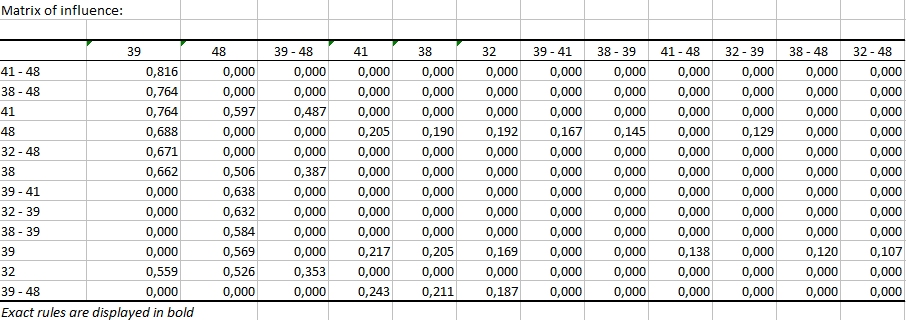The chart of influence is the graphical view of the same table.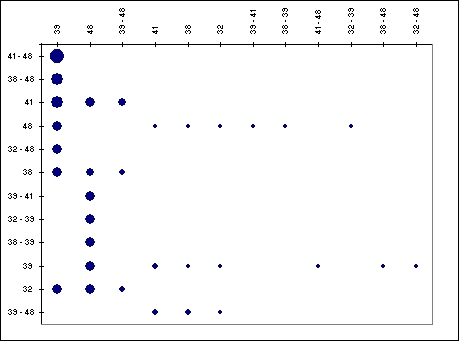The next table is a symmetric matrix that averages the confidences between products involved in rules that meet the criteria of minimum support and confidence. This table is then used to create a visualization of the products based on their proximities (based on MDS method, a unique feature developed by Addinsoft in 2014).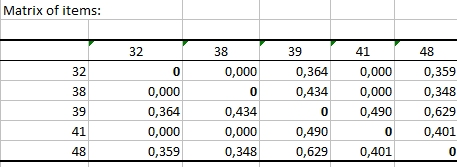On the items chart we see that products 48 and 39 are the closest.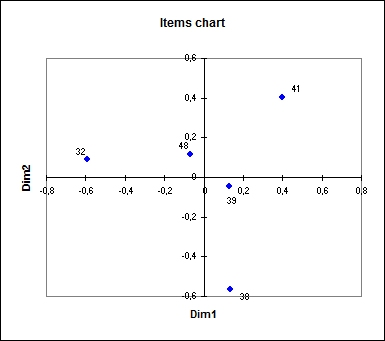The approach developed here shows how to use association rules to analyze consumer baskets. The interpretation and operational decisions that arise from these results highly depend on the knowledge that one has of the market and the products.

1c26995d494fb3061dd0ae8571ffc0a4@xlstat.desk-mail.com
https://cdn.desk.com/
false
desk